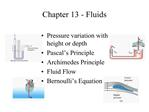# Chapter 13 - Fluids - PowerPoint PPT PresentationDownload PresentationChapter 13 - Fluids

Chapter 13 - Fluids
Download Presentation## Chapter 13 - Fluids

- - - - - - - - - - - - - - - - - - - - - - - - - - - E N D - - - - - - - - - - - - - - - - - - - - - - - - - - -
##### Presentation Transcript

1. Chapter 13 - Fluids Pressure variation with height or depth Pascal�s Principle Archimedes Principle Fluid Flow Bernoulli�s Equation

3. Density Which is heavier, wood or iron? Amount of mass per unit volume Compressible - Density easily altered Incompressible - Density varies only slightly or not at all. Specific Gravity - ratio of the density of a material to that of water

4. Pressure The normal force per unit area that a fluid exerts on the walls of its container, adjacent fluids or other boundaries. Units: N/m2 = 1 Pascal 1 Bar = 105 Pa 1 atm = 1.013 x 105 Pa 1 atm = 14.7 lb/in2 1 atm = 760 Torr (mm Hg)

5. Variation of pressure with height (depth)

6. Variation of pressure with height (depth)

7. Problem 1 � What is the pressure at the faucet?

8. Absolute vs. Gauge Pressure Gauge Pressure That read on a gauge which compares it to atmospheric pressure Absolute Pressure Sum of the gauge pressure and atmospheric pressure

9. Problem 2 What is the pressure at a depth of 1300 feet (approximately 400 m) rw = 1000 kg/m3

10. Problem 3 What is the height of a mercury column if the pressure at the bottom is 101.325 kPa and the pressure at the top is zero? rHg = 13.595 x 103 kg/m3

11. Pascal�s Principle An increase in the pressure at any point in a confined fluid is transmitted undiminished throughout the fluid volume and to the walls of the container.

12. Problem 4 In the system below, a 1.0 N force is applied to the piston at the left. The piston is moved 5.0 cm What is the force on the large mass on the right. How far can the large block move?

13. Archimedes Principle The buoyancy force on an object is equal to the weight of the fluid it displaces. Pressure at the top of an object is less than at the bottom

14. Problem 5 What volume of water must be displaced for a 6900 Ton submarine to hover?

15. Fluid dynamics - equation of continuity Laminar flow Mass flow rate is constant

16. Problem 4 Water leaves the nozzle of a firehose at 50 m/s. What is the velocity of water in the hose. Hose inner diameter = 10 cm Nozzle inner diameter = 3 cm Using the rocket equation, how much thrust does a fireman feel from this exiting fluid?

17. Bernoulli�s principal Where the velocity of a fluid is high, the pressure of a fluid is low, and where the velocity is low, the pressure is high.

18. Bernoulli�s equation

19. Bernoulli�s equation

20. Problem 5 Find the pressure in the fire hose with exhaust velocity = 50 m/s

21. Problem 6 What is the velocity of the fluid leaving the pipe at point 2. A small pipe directs the water upward. What is the height of the plume?

22. Problem 7 (#47) What gauge pressure is necessary in a fire main if a firehose is to spray water to a height of 15 m?

23. Problem 8 (#50) If the wind blows at 25 m/s over your house, what is the net force on the roof if its area is 240 m2?